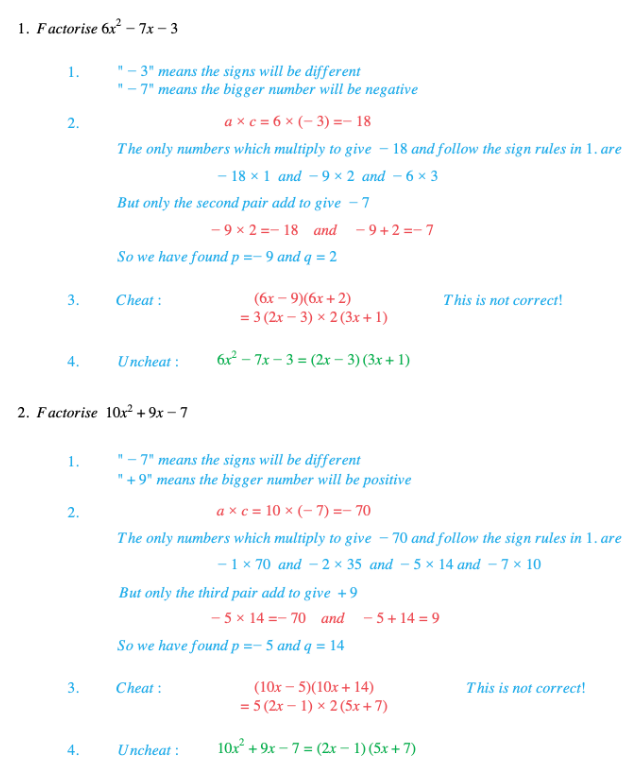# Edexcel IGCSE Maths 复习笔记 2.4.2 Factorising Quadratics - Harder

Edexcel IGCSE Maths 复习笔记 2.4.2 Factorising Quadratics - Harder

#### What is a quadratic expression?

• A quadratic expression looks like this:ax2 + bx + c (as long as a ≠ 0)
• Note: If there are any higher powers of x (like x3 say) then it is not a quadratic!

#### Factorising a 3 term quadratic expression (a ≠ 1)

• Signs in quadratic determine signs in brackets:
• If c is positive then both signs are the same as the sign of b
• If c is negative then the signs are different and bigger number has the sign of b

• Using those signs find numbers p and q which
• Multiply to give a × c
• Cheat by writing brackets as (ax + p)(ax + q) – Yes, I know! This is not correct!
• Uncheat by cancelling common factors in each bracket

#### Exam Tip

Check for a common factor – life will be a lot easier if you take it out before you try to factorise.If a is negative take out out a factor of -1 – that will help, too.

#### Worked Example